## 9.6 Conclusion

### 9.6.1 Theory-based hypothesis tests

Much as we did in Subsection 8.7.2 when we showed you a theory-based method for constructing confidence intervals that involved mathematical formulas, we now present an example of a traditional theory-based method to conduct hypothesis tests. This method relies on probability models, probability distributions, and a few assumptions to construct the null distribution. This is in contrast to the approach we’ve been using throughout this book where we relied on computer simulations to construct the null distribution.

These traditional theory-based methods have been used for decades mostly because researchers didn’t have access to computers that could run thousands of calculations quickly and efficiently. Now that computing power is much cheaper and more accessible, simulation-based methods are much more feasible. However, researchers in many fields continue to use theory-based methods. Hence, we make it a point to include an example here.

As we’ll show in this section, any theory-based method is ultimately an approximation to the simulation-based method. The theory-based method we’ll focus on is known as the two-sample $$t$$-test for testing differences in sample means. However, the test statistic we’ll use won’t be the difference in sample means $$\overline{x}_1 - \overline{x}_2$$, but rather the related two-sample $$t$$-statistic. The data we’ll use will once again be the movies_sample data of action and romance movies from Section 9.5.

#### Two-sample t-statistic

A common task in statistics is the process of “standardizing a variable.” By standardizing different variables, we make them more comparable. For example, say you are interested in studying the distribution of temperature recordings from Portland, Oregon, USA and comparing it to that of the temperature recordings in Montreal, Quebec, Canada. Given that US temperatures are generally recorded in degrees Fahrenheit and Canadian temperatures are generally recorded in degrees Celsius, how can we make them comparable? One approach would be to convert degrees Fahrenheit into Celsius, or vice versa. Another approach would be to convert them both to a common “standardized” scale, like Kelvin units of temperature.

One common method for standardizing a variable from probability and statistics theory is to compute the $$z$$-score:

$z = \frac{x - \mu}{\sigma}$

where $$x$$ represents one value of a variable, $$\mu$$ represents the mean of that variable, and $$\sigma$$ represents the standard deviation of that variable. You first subtract the mean $$\mu$$ from each value of $$x$$ and then divide $$x - \mu$$ by the standard deviation $$\sigma$$. These operations will have the effect of re-centering your variable around 0 and re-scaling your variable $$x$$ so that they have what are known as “standard units.” Thus for every value that your variable can take, it has a corresponding $$z$$-score that gives how many standard units away that value is from the mean $$\mu$$. $$z$$-scores are normally distributed with mean 0 and standard deviation 1. This curve is called a “$$z$$-distribution” or “standard normal” curve and has the common, bell-shaped pattern from Figure 9.19 discussed in Appendix A.2.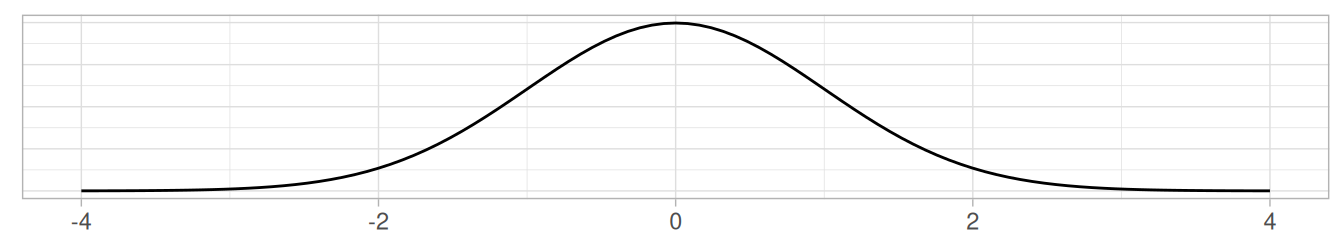FIGURE 9.19: Standard normal z curve.

Bringing these back to the difference of sample mean ratings $$\overline{x}_a - \overline{x}_r$$ of action versus romance movies, how would we standardize this variable? By once again subtracting its mean and dividing by its standard deviation. Recall two facts from Subsection 7.3.3. First, if the sampling was done in a representative fashion, then the sampling distribution of $$\overline{x}_a - \overline{x}_r$$ will be centered at the true population parameter $$\mu_a - \mu_r$$. Second, the standard deviation of point estimates like $$\overline{x}_a - \overline{x}_r$$ has a special name: the standard error.

Applying these ideas, we present the two-sample $$t$$-statistic:

$t = \dfrac{ (\bar{x}_a - \bar{x}_r) - (\mu_a - \mu_r)}{ \text{SE}_{\bar{x}_a - \bar{x}_r} } = \dfrac{ (\bar{x}_a - \bar{x}_r) - (\mu_a - \mu_r)}{ \sqrt{\dfrac{{s_a}^2}{n_a} + \dfrac{{s_r}^2}{n_r}} }$

Oofda! There is a lot to try to unpack here! Let’s go slowly. In the numerator, $$\bar{x}_a-\bar{x}_r$$ is the difference in sample means, while $$\mu_a - \mu_r$$ is the difference in population means. In the denominator, $$s_a$$ and $$s_r$$ are the sample standard deviations of the action and romance movies in our sample movies_sample. Lastly, $$n_a$$ and $$n_r$$ are the sample sizes of the action and romance movies. Putting this together under the square root gives us the standard error $$\text{SE}_{\bar{x}_a - \bar{x}_r}$$.

Observe that the formula for $$\text{SE}_{\bar{x}_a - \bar{x}_r}$$ has the sample sizes $$n_a$$ and $$n_r$$ in them. So as the sample sizes increase, the standard error goes down. We’ve seen this concept numerous times now, in particular in our simulations using the three virtual shovels with $$n$$ = 25, 50, and 100 slots in Figure 7.15 and in Subsection 8.5.3 where we studied the effect of using larger sample sizes on the widths of confidence intervals.

So how can we use the two-sample $$t$$-statistic as a test statistic in our hypothesis test? First, assuming the null hypothesis $$H_0: \mu_a - \mu_r = 0$$ is true, the right-hand side of the numerator (to the right of the $$-$$ sign), $$\mu_a - \mu_r$$, becomes 0.

Second, similarly to how the Central Limit Theorem from Subsection 7.5.2 states that sample means follow a normal distribution, it can be mathematically proven that the two-sample $$t$$-statistic follows a $$t$$ distribution with degrees of freedom “roughly equal” to $$df = n_a + n_r - 2$$. To better understand this concept of degrees of freedom, we next display three examples of $$t$$-distributions in Figure 9.20 along with the standard normal $$z$$ curve.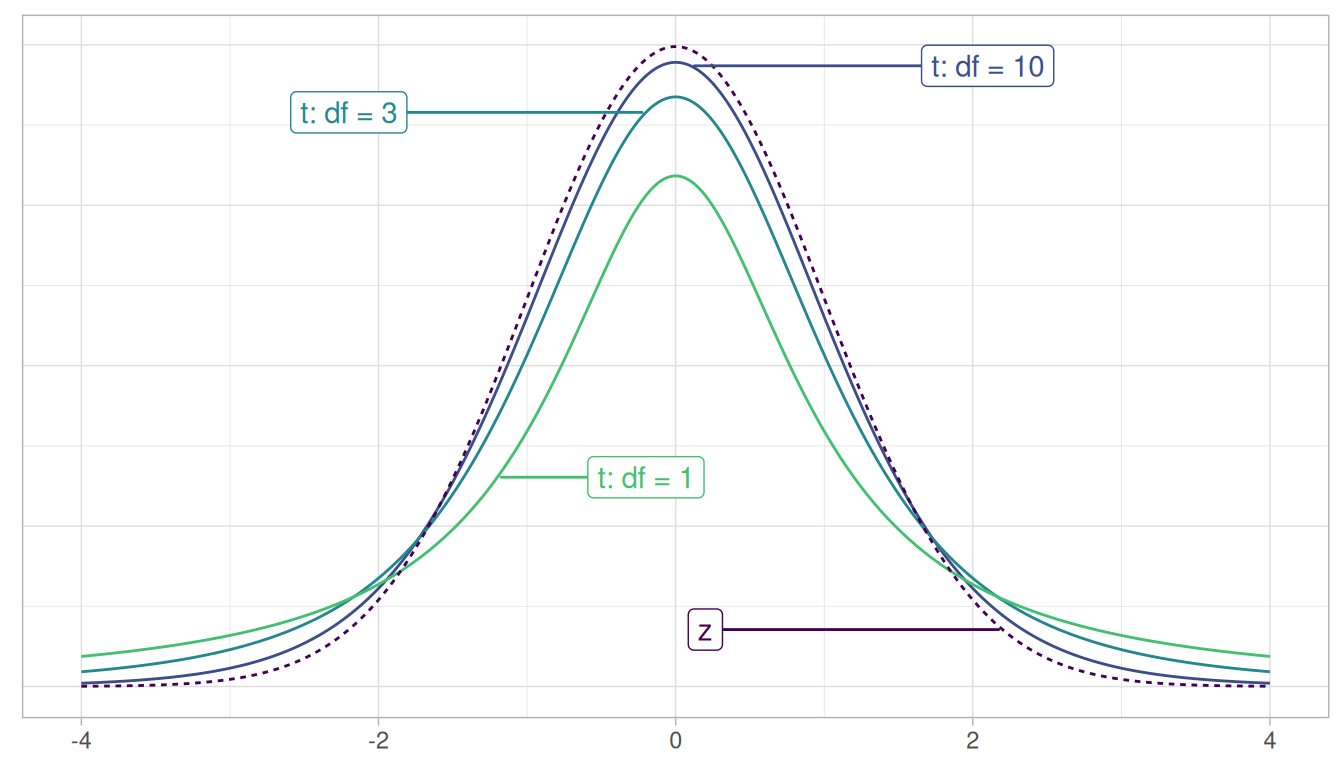FIGURE 9.20: Examples of t-distributions and the z curve.

Begin by looking at the center of the plot at 0 on the horizontal axis. As you move up from the value of 0, follow along with the labels and note that the bottom curve corresponds to 1 degree of freedom, the curve above it is for 3 degrees of freedom, the curve above that is for 10 degrees of freedom, and lastly the dotted curve is the standard normal $$z$$ curve.

Observe that all four curves have a bell shape, are centered at 0, and that as the degrees of freedom increase, the $$t$$-distribution more and more resembles the standard normal $$z$$ curve. The “degrees of freedom” measures how different the $$t$$ distribution will be from a normal distribution. $$t$$-distributions tend to have more values in the tails of their distributions than the standard normal $$z$$ curve.

This “roughly equal” statement indicates that the equation $$df = n_a + n_r - 2$$ is a “good enough” approximation to the true degrees of freedom. The true formula is a bit more complicated than this simple expression, but we’ve found the formula to be beyond the reach of those new to statistical inference and it does little to build the intuition of the $$t$$-test.

The message to retain, however, is that small sample sizes lead to small degrees of freedom and thus small sample sizes lead to $$t$$-distributions that are different than the $$z$$ curve. On the other hand, large sample sizes correspond to large degrees of freedom and thus produce $$t$$ distributions that closely align with the standard normal $$z$$-curve.

So, assuming the null hypothesis $$H_0$$ is true, our formula for the test statistic simplifies a bit:

$t = \dfrac{ (\bar{x}_a - \bar{x}_r) - 0}{ \sqrt{\dfrac{{s_a}^2}{n_a} + \dfrac{{s_r}^2}{n_r}} } = \dfrac{ \bar{x}_a - \bar{x}_r}{ \sqrt{\dfrac{{s_a}^2}{n_a} + \dfrac{{s_r}^2}{n_r}} }$

Let’s compute the values necessary for this two-sample $$t$$-statistic. Recall the summary statistics we computed during our exploratory data analysis in Section 9.5.1.

movies_sample %>%
group_by(genre) %>%
summarize(n = n(), mean_rating = mean(rating), std_dev = sd(rating))
# A tibble: 2 x 4
genre       n mean_rating std_dev
<chr>   <int>       <dbl>   <dbl>
1 Action     32     5.275   1.36121
2 Romance    36     6.32222 1.60963

Using these values, the observed two-sample $$t$$-test statistic is

$\dfrac{ \bar{x}_a - \bar{x}_r}{ \sqrt{\dfrac{{s_a}^2}{n_a} + \dfrac{{s_r}^2}{n_r}} } = \dfrac{5.28 - 6.32}{ \sqrt{\dfrac{{1.36}^2}{32} + \dfrac{{1.61}^2}{36}} } = -2.906$

Great! How can we compute the $$p$$-value using this theory-based test statistic? We need to compare it to a null distribution, which we construct next.

#### Null distribution

Let’s revisit the null distribution for the test statistic $$\bar{x}_a - \bar{x}_r$$ we constructed in Section 9.5. Let’s visualize this in the left-hand plot of Figure 9.21.

# Construct null distribution of xbar_a - xbar_r:
null_distribution_movies <- movies_sample %>%
specify(formula = rating ~ genre) %>%
hypothesize(null = "independence") %>%
generate(reps = 1000, type = "permute") %>%
calculate(stat = "diff in means", order = c("Action", "Romance"))
visualize(null_distribution_movies, bins = 10)

The infer package also includes some built-in theory-based test statistics as well. So instead of calculating the test statistic of interest as the "diff in means" $$\bar{x}_a - \bar{x}_r$$, we can calculate this defined two-sample $$t$$-statistic by setting stat = "t". Let’s visualize this in the right-hand plot of Figure 9.21.

# Construct null distribution of t:
null_distribution_movies_t <- movies_sample %>%
specify(formula = rating ~ genre) %>%
hypothesize(null = "independence") %>%
generate(reps = 1000, type = "permute") %>%
# Notice we switched stat from "diff in means" to "t"
calculate(stat = "t", order = c("Action", "Romance"))
visualize(null_distribution_movies_t, bins = 10)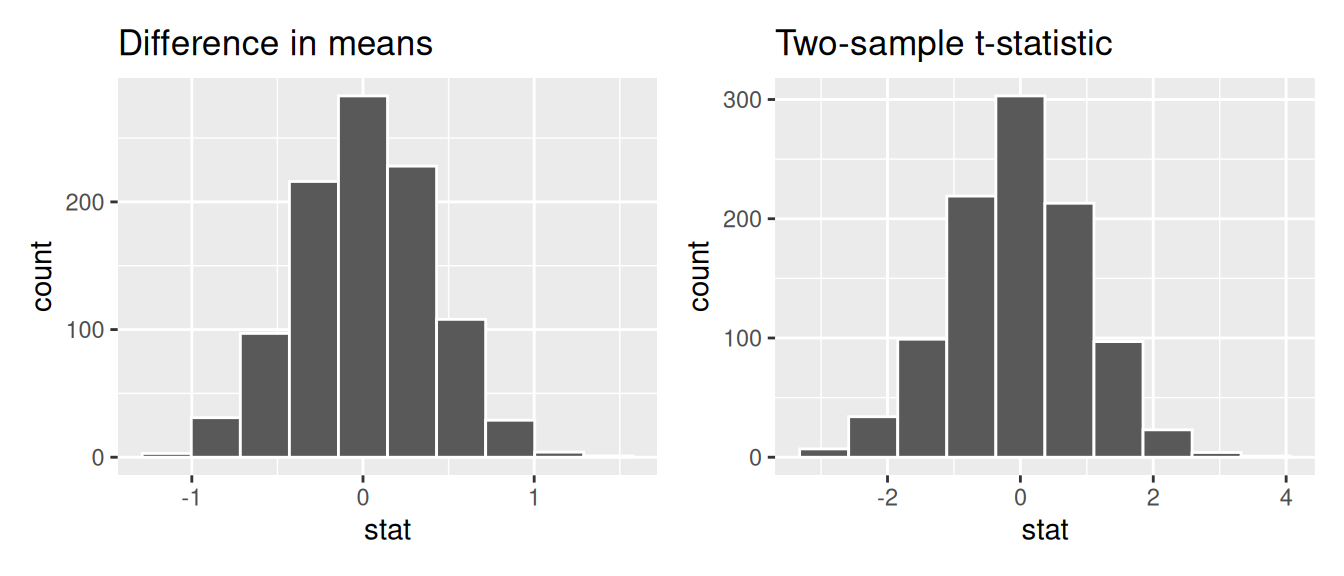FIGURE 9.21: Comparing the null distributions of two test statistics.

Observe that while the shape of the null distributions of both the difference in means $$\bar{x}_a - \bar{x}_r$$ and the two-sample $$t$$-statistics are similar, the scales on the x-axis are different. The two-sample $$t$$-statistic values are spread out over a larger range.

However, a traditional theory-based $$t$$-test doesn’t look at the simulated histogram in null_distribution_movies_t, but instead it looks at the $$t$$-distribution curve with degrees of freedom equal to roughly 65.85. This calculation is based on the complicated formula referenced previously, which we approximated with $$df = n_a + n_r - 2 = 32 + 36 - 2 = 66$$. Let’s overlay this $$t$$-distribution curve over the top of our simulated two-sample $$t$$-statistics using the method = "both" argument in visualize().

visualize(null_distribution_movies_t, bins = 10, method = "both")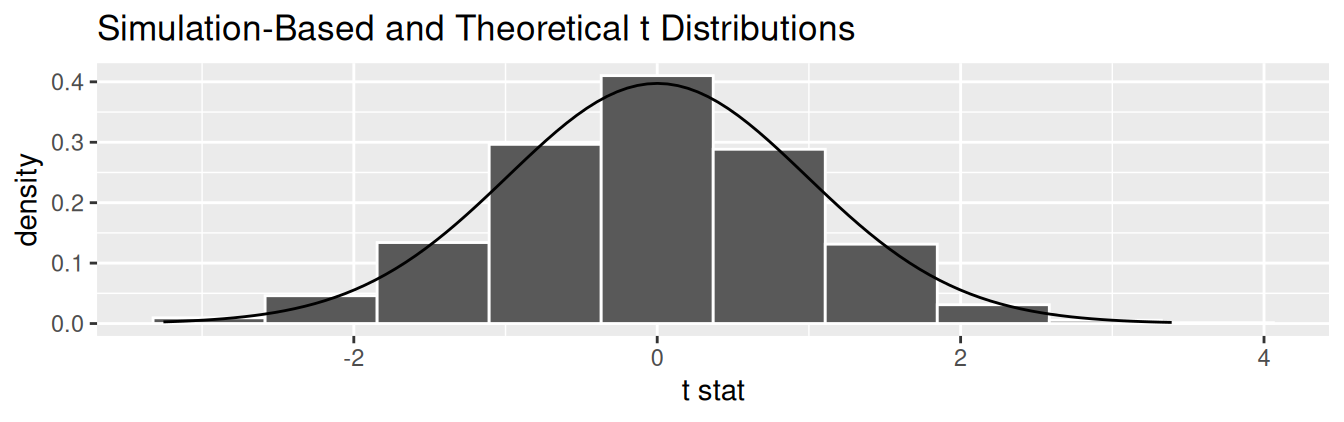FIGURE 9.22: Null distribution using t-statistic and t-distribution.

Observe that the curve does a good job of approximating the histogram here. To calculate the $$p$$-value in this case, we need to figure out how much of the total area under the $$t$$-distribution curve is at or “more extreme” than our observed two-sample $$t$$-statistic. Since $$H_A: \mu_a - \mu_r \neq 0$$ is a two-sided alternative, we need to add up the areas in both tails.

We first compute the observed two-sample $$t$$-statistic using infer verbs. This shortcut calculation further assumes that the null hypothesis is true: that the population of action and romance movies have an equal average rating.

obs_two_sample_t <- movies_sample %>%
specify(formula = rating ~ genre) %>%
calculate(stat = "t", order = c("Action", "Romance"))
obs_two_sample_t
# A tibble: 1 x 1
stat
<dbl>
1 -2.90589

We want to find the percentage of values that are at or below obs_two_sample_t $$= -2.906$$ or at or above -obs_two_sample_t $$= 2.906$$. We use the shade_p_value() function with the direction argument set to "both" to do this:

visualize(null_distribution_movies_t, method = "both") +
shade_p_value(obs_stat = obs_two_sample_t, direction = "both")
Warning: Check to make sure the conditions have been met for the theoretical
method. {infer} currently does not check these for you.FIGURE 9.23: Null distribution using t-statistic and t-distribution with $$p$$-value shaded.

(We’ll discuss this warning message shortly.) What is the $$p$$-value? We apply get_p_value() to our null distribution saved in null_distribution_movies_t:

null_distribution_movies_t %>%
get_p_value(obs_stat = obs_two_sample_t, direction = "both")
# A tibble: 1 x 1
p_value
<dbl>
1   0.002

We have a very small $$p$$-value, and thus it is very unlikely that these results are due to sampling variation. Thus, we are inclined to reject $$H_0$$.

Let’s come back to that earlier warning message: Check to make sure the conditions have been met for the theoretical method. {infer} currently does not check these for you. To be able to use the $$t$$-test and other such theoretical methods, there are always a few conditions to check. The infer package does not automatically check these conditions, hence the warning message we received. These conditions are necessary so that the underlying mathematical theory holds. In order for the results of our two-sample $$t$$-test to be valid, three conditions must be met:

1. Nearly normal populations or large sample sizes. A general rule of thumb that works in many (but not all) situations is that the sample size $$n$$ should be greater than 30.
2. Both samples are selected independently of each other.
3. All observations are independent from each other.

Let’s see if these conditions hold for our movies_sample data:

1. This is met since $$n_a$$ = 32 and $$n_r$$ = 36 are both larger than 30, satisfying our rule of thumb.
2. This is met since we sampled the action and romance movies at random and in an unbiased fashion from the database of all IMDb movies.
3. Unfortunately, we don’t know how IMDb computes the ratings. For example, if the same person rated multiple movies, then those observations would be related and hence not independent.

Assuming all three conditions are roughly met, we can be reasonably certain that the theory-based $$t$$-test results are valid. If any of the conditions were clearly not met, we couldn’t put as much trust into any conclusions reached. On the other hand, in most scenarios, the only assumption that needs to be met in the simulation-based method is that the sample is selected at random. Thus, in our experience, we prefer simulation-based methods as they have fewer assumptions, are conceptually easier to understand, and since computing power has recently become easily accessible, they can be run quickly. That being said since much of the world’s research still relies on traditional theory-based methods, we also believe it is important to understand them.

You may be wondering why we chose reps = 1000 for these simulation-based methods. We’ve noticed that after around 1000 replicates for the null distribution and the bootstrap distribution for most problems you can start to get a general sense for how the statistic behaves. You can change this value to something like 10,000 though for reps if you would like even finer detail but this will take more time to compute. Feel free to iterate on this as you like to get an even better idea about the shape of the null and bootstrap distributions as you wish.

### 9.6.2 When inference is not needed

We’ve now walked through several different examples of how to use the infer package to perform statistical inference: constructing confidence intervals and conducting hypothesis tests. For each of these examples, we made it a point to always perform an exploratory data analysis (EDA) first; specifically, by looking at the raw data values, by using data visualization with ggplot2, and by data wrangling with dplyr beforehand. We highly encourage you to always do the same. As a beginner to statistics, EDA helps you develop intuition as to what statistical methods like confidence intervals and hypothesis tests can tell us. Even as a seasoned practitioner of statistics, EDA helps guide your statistical investigations. In particular, is statistical inference even needed?

Let’s consider an example. Say we’re interested in the following question: Of all flights leaving a New York City airport, are Hawaiian Airlines flights in the air for longer than Alaska Airlines flights? Furthermore, let’s assume that 2013 flights are a representative sample of all such flights. Then we can use the flights data frame in the nycflights13 package we introduced in Section 1.4 to answer our question. Let’s filter this data frame to only include Hawaiian and Alaska Airlines using their carrier codes HA and AS:

flights_sample <- flights %>%
filter(carrier %in% c("HA", "AS"))

There are two possible statistical inference methods we could use to answer such questions. First, we could construct a 95% confidence interval for the difference in population means $$\mu_{HA} - \mu_{AS}$$, where $$\mu_{HA}$$ is the mean air time of all Hawaiian Airlines flights and $$\mu_{AS}$$ is the mean air time of all Alaska Airlines flights. We could then check if the entirety of the interval is greater than 0, suggesting that $$\mu_{HA} - \mu_{AS} > 0$$, or, in other words suggesting that $$\mu_{HA} > \mu_{AS}$$. Second, we could perform a hypothesis test of the null hypothesis $$H_0: \mu_{HA} - \mu_{AS} = 0$$ versus the alternative hypothesis $$H_A: \mu_{HA} - \mu_{AS} > 0$$.

However, let’s first construct an exploratory visualization as we suggested earlier. Since air_time is numerical and carrier is categorical, a boxplot can display the relationship between these two variables, which we display in Figure 9.24.

ggplot(data = flights_sample, mapping = aes(x = carrier, y = air_time)) +
geom_boxplot() +
labs(x = "Carrier", y = "Air Time")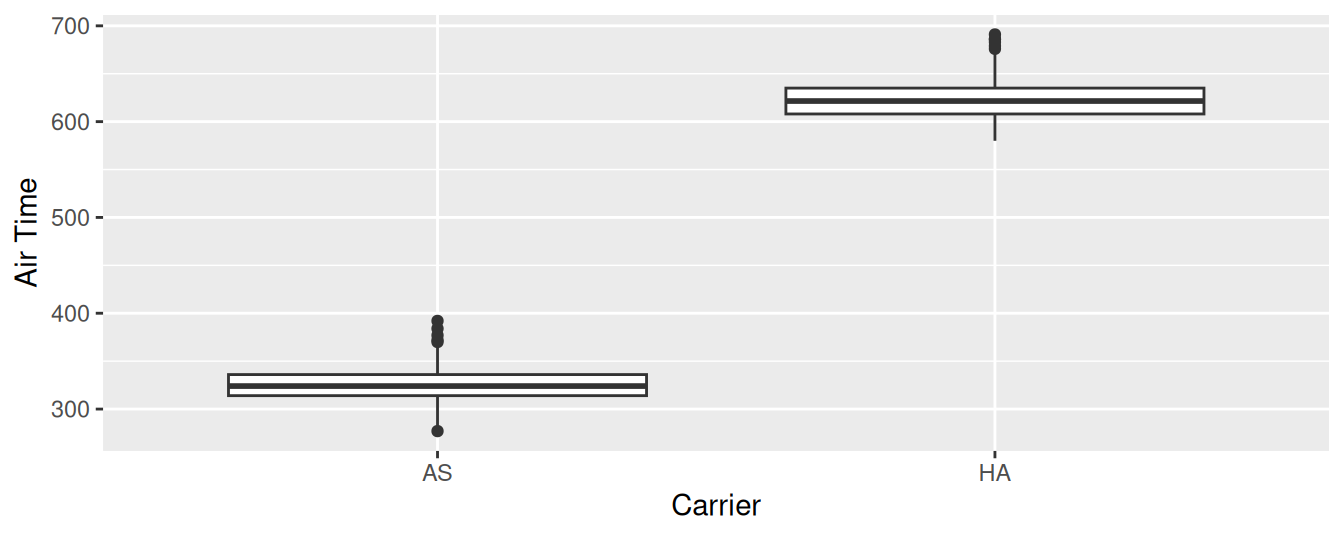FIGURE 9.24: Air time for Hawaiian and Alaska Airlines flights departing NYC in 2013.

This is what we like to call “no PhD in Statistics needed” moments. You don’t have to be an expert in statistics to know that Alaska Airlines and Hawaiian Airlines have significantly different air times. The two boxplots don’t even overlap! Constructing a confidence interval or conducting a hypothesis test would frankly not provide much more insight than Figure 9.24.

Let’s investigate why we observe such a clear cut difference between these two airlines using data wrangling. Let’s first group by the rows of flights_sample not only by carrier but also by destination dest. Subsequently, we’ll compute two summary statistics: the number of observations using n() and the mean airtime:

flights_sample %>%
group_by(carrier, dest) %>%
summarize(n = n(), mean_time = mean(air_time, na.rm = TRUE))
# A tibble: 2 x 4
# Groups:   carrier 
carrier dest      n mean_time
<chr>   <chr> <int>     <dbl>
1 AS      SEA     714   325.618
2 HA      HNL     342   623.088

It turns out that from New York City in 2013, Alaska only flew to SEA (Seattle) from New York City (NYC) while Hawaiian only flew to HNL (Honolulu) from NYC. Given the clear difference in distance from New York City to Seattle versus New York City to Honolulu, it is not surprising that we observe such different (statistically significantly different, in fact) air times in flights.

This is a clear example of not needing to do anything more than a simple exploratory data analysis using data visualization and descriptive statistics to get an appropriate conclusion. This is why we highly recommend you perform an EDA of any sample data before running statistical inference methods like confidence intervals and hypothesis tests.

### 9.6.3 Problems with p-values

On top of the many common misunderstandings about hypothesis testing and $$p$$-values we listed in Section 9.4, another unfortunate consequence of the expanded use of $$p$$-values and hypothesis testing is a phenomenon known as “p-hacking.” p-hacking is the act of “cherry-picking” only results that are “statistically significant” while dismissing those that aren’t, even if at the expense of the scientific ideas. There are lots of articles written recently about misunderstandings and the problems with $$p$$-values. We encourage you to check some of them out:

Such issues were getting so problematic that the American Statistical Association (ASA) put out a statement in 2016 titled, “The ASA Statement on Statistical Significance and $$P$$-Values,” with six principles underlying the proper use and interpretation of $$p$$-values. The ASA released this guidance on $$p$$-values to improve the conduct and interpretation of quantitative science and to inform the growing emphasis on reproducibility of science research.

We as authors much prefer the use of confidence intervals for statistical inference, since in our opinion they are much less prone to large misinterpretation. However, many fields still exclusively use $$p$$-values for statistical inference and this is one reason for including them in this text. We encourage you to learn more about “p-hacking” as well and its implication for science.

An R script file of all R code used in this chapter is available here.

If you want more examples of the infer workflow for conducting hypothesis tests, we suggest you check out the infer package homepage, in particular, a series of example analyses available at https://infer.netlify.app/articles/.

### 9.6.5 What’s to come

We conclude with the infer pipeline for hypothesis testing in Figure 9.25.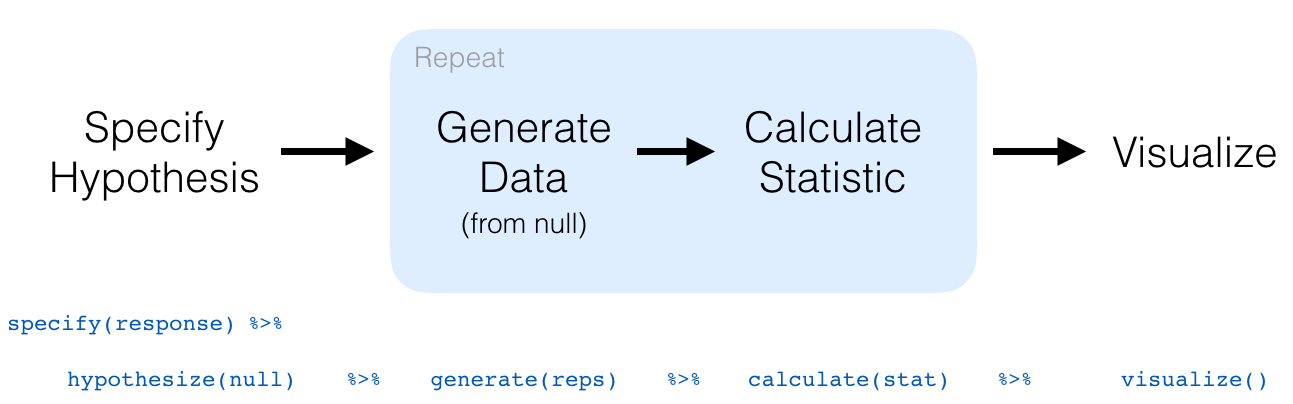FIGURE 9.25: infer package workflow for hypothesis testing.

Now that we’ve armed ourselves with an understanding of confidence intervals from Chapter 8 and hypothesis tests from this chapter, we’ll now study inference for regression in the upcoming Chapter 10.

We’ll revisit the regression models we studied in Chapter 5 on basic regression and Chapter 6 on multiple regression. For example, recall Table 5.2 (shown again here in Table 9.4), corresponding to our regression model for an instructor’s teaching score as a function of their “beauty” score.

# Fit regression model:
score_model <- lm(score ~ bty_avg, data = evals)

# Get regression table:
get_regression_table(score_model)
TABLE 9.4: Linear regression table
term estimate std_error statistic p_value lower_ci upper_ci
intercept 3.880 0.076 50.96 0 3.731 4.030
bty_avg 0.067 0.016 4.09 0 0.035 0.099

We previously saw in Subsection 5.1.2 that the values in the estimate column are the fitted intercept $$b_0$$ and fitted slope for beauty score $$b_1$$. In Chapter 10, we’ll unpack the remaining columns: std_error which is the standard error, statistic which is the observed standardized test statistic to compute the p_value, and the 95% confidence intervals as given by lower_ci and upper_ci.